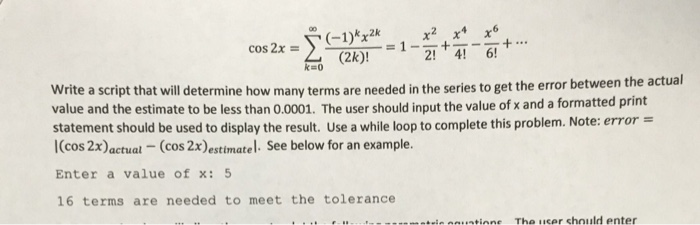Homework Help Question & Answers

X) -1-2! +41-614 (2k)! k=0 he actual Write a script that will determine how many terms are needed...x) -1-2! +41-614 (2k)! k=0 he actual Write a script that will determine how many terms are needed in the series to get the error between t value and the estimate to be less than 0.0001. The user should input the value of x and a formatted print statement should be used to display the result. Use a while loop to complete this problem. Note: error I(cos 2x)actual - (cos 2x)estimatel. See below for an example. Enter a value of x: 5 16 terms are needed to meet the tolerance

MATLAB CODE---------------------------

x=input('Enter value of x');

x1=(x*pi)/180;

COS2x_Actual=cosd(2*x)

n=0;

Error=1;

COS2X_estimated=0;

while (Error>0.0001)

COS2X_estimated =COS2X_estimated +(((-1)^(n)*((2*x1)^(2*n)))/factorial(2*n))

Error = abs(COS2x_Actual-COS2X_estimated)

n=n+1;

end

fprintf('\n\n%d terms are needed to meet the tolerance',n)

OUTPUT----------------TEST---------------------OF-----------CODE

Enter value of x> 45
COS2x_Actual = 0
COS2X_estimated =  1
Error =  1
COS2X_estimated = -0.23370
Error =  0.23370
COS2X_estimated =  0.019969
Error =  0.019969
COS2X_estimated =   -8.9452e-04
Error =    8.9452e-04
COS2X_estimated =    2.4737e-05
Error =    2.4737e-05

5 terms are needed to meet the tolerance

Enter value of x> 5
COS2x_Actual =  0.98481
COS2X_estimated =  1
Error =  0.015192
COS2X_estimated =  0.98477
Error =    3.8624e-05

2 terms are needed to meet the tolerance
Add Answer of: X) -1-2! +41-614 (2k)! k=0 he actual Write a script that will determine how many terms are needed...
More Homework Help Questions Additional questions in this topic.

• Find the values of the missing items. Assume that the actual sales volume equals actual productio...

Need Online Homework Help?

Get FREE EXPERT Answers
WITHIN MINUTES
Related Questions